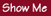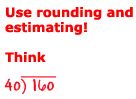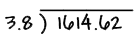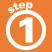Home    |    Teacher    |    Parents    |    Glossary    |    About UsTo divide decimal numbers:

1. If the divisor is not a whole number, move decimal point to right to make it a whole number and move decimal point in dividend the same number of places.2. Divide as usual. Keep dividing until the answer terminates or repeats.3. Put decimal point directly above decimal point in the dividend.4. Check your answer. Multiply quotient by divisor. Does it equal the dividend?Homework Help | Pre-Algebra | NumbersEmail this page to a friendSearch·  Place value·  Decimal numbers·  Estimating and     rounding·  Adding / subtracting     decimals·  Multiplying decimals·  Dividing decimals·  Percent·  Exponents·  Square roots·  Signed integers·  Adding and     subtracting integers·  Multiplying and     dividing integers·  Properties of integersFirst Glance In Depth Examples WorkoutDividing decimals